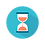From: Bayesian Models for Astrophysical Data, Cambridge Univ. Press

(c) 2017,  Joseph M. Hilbe, Rafael S. de Souza and Emille E. O. Ishida

you are kindly asked to include the complete citation if you used this material in a publication

# Data from code 5.15
set.seed(13979)
nobs <- 5000

x1 <- rbinom(nobs, size = 1, 0.6)
x2 <- runif(nobs)
xb <- 2 + 0.75*x1 - 5*x2

exb <- 1/(1+exp(-xb))
by <- rbinom(nobs, size = 1, prob = exb)

logitmod <- data.frame(by, x1, x2)

Code 5.17 Logistic model in R using JAGS

====================================================

attach(logitmod)
require(R2jags)

X <- model.matrix(~ x1 + x2,
data = logitmod)

K <- ncol(X)

model.data <- list(Y = logitmod\$by,
N = nrow(logitmod),
X = X,
K = K,
LogN = log(nrow(logitmod)),
b0 = rep(0, K),
B0 = diag(0.00001, K)
)

sink("LOGIT.txt")

cat("
model{
# Priors
beta ~ dmnorm(b0[], B0[,])

# Likelihood
for (i in 1:N){
Y[i] ~ dbern(p[i])
logit(p[i]) <- eta[i]

# logit(p[i]) <- max(-20,min(20,eta[i])) used to avoid numerical instabilities
# p[i] <- 1/(1+exp(-eta[i])) can use for logit(p[i]) above

eta[i] <- inprod(beta[], X[i,])
LLi[i] <- Y[i] * log(p[i]) + (1 - Y[i]) * log(1 - p[i])
}

LogL <- sum(LLi[1:N])
AIC <- -2 * LogL + 2 * K
BIC <- -2 * LogL + LogN * K
}
"
,fill = TRUE)

sink()

# Initial parameter values
inits <- function () {
list(beta = rnorm(K, 0, 0.1)) }

params <- c("beta", "LogL", "AIC", "BIC")

LOGT <- jags(data = model.data,
inits = inits,
parameters = params,
model.file = "LOGIT.txt",
n.thin = 1,
n.chains = 3,
n.burnin = 5000,
n.iter = 10000)

print(LOGT, intervals=c(0.025, 0.975), digits=3)

====================================================Output on screen:

Inference for Bugs model at "LOGIT.txt", fit using jags,

3 chains, each with 10000 iterations (first 5000 discarded)

n.sims = 15000 iterations saved

mu.vect      sd.vect             2.5%           97.5%        Rhat         n.eff

AIC           5080.329        2.492       5077.514      5086.838       1.002        1300

BIC           5099.881        2.492       5097.065      5106.390       1.002        1300

LogL       -2537.165        1.246      -2540.419     -2535.757       1.002        1300

beta            1.948        0.085             1.780            2.111        1.006         360

beta            0.849        0.072             0.705            0.991        1.002       2400

beta           -5.018        0.149           -5.308           -4.724        1.004         570

deviance   5074.329        2.492       5071.514      5080.838        1.002       1300

For each parameter, n.eff is a crude measure of effective sample size,

and Rhat is the potential scale reduction factor (at convergence, Rhat=1).

DIC info (using the rule, pD = var(deviance)/2)

pD = 3.1 and DIC = 5077.4

DIC is an estimate of expected predictive error (lower deviance is better).